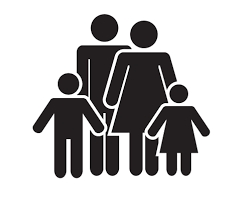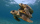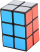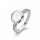# Timothy

Timothy is 56 4/5 inches tall. Theresa is 1 1/6 inches shorter than Timothy and Jane is 1 1/3 inches shorter than Theresa. How tall is Jane?

J =  54.3 in

### Step-by-step explanation:Did you find an error or inaccuracy? Feel free to write us. Thank you!Tips to related online calculators
Do you want to convert length units?

## Related math problems and questions:

• Thomas 2Thomas is 56 3/4 inches tall. Jillian is 1 3/8 inches shorter than Thomas and Jane is 1 1/5 inches shorter than Jillian. How tall is Jane? Label the names on the tape diagram to represent this problem. Jane Jillian Thomas Excellent!
• Max vs SharonMax is 1 1/2 times as tall as Sharon. Alan is 4 1/3 feet tall. If Alan is 6 inches taller than Sharon, how tall is Max?
• A turtleA turtle is 20 5/6 inches below the surface of a pond. It dives to a depth of 32 1/4 inches. What is the change in the turtle’s position? Write your answer as a mixed number.
• Metal rodYou have a metal rod that’s 51/64 inches long. The rod needs to be trimmed. You cut 1/64 inches from one end and 1/32 inches from the other end. Next, you cut the rod into 6 equal pieces. What will be the final length of each piece?A gift box has dimensions of: 8 1/2 inches, 5 1/2 inches, and 2 1/2 inches, respectively. How many cubes with side lengths of 1/2 inches would be needed to fill the gift box?
• TreesA young tree is 16 inches tall. One year later, it is 20 inches tall. What is the percent increase in height?
• A rectangle 6A rectangle measures 2 4/5 inches by 2 1/5 inches. What is its area?
• Jewelry boxThe bottom of Zeyda's jewelry box is a rectangle with the length of 5 3/8 inches and a width of 3 1/4 inches. What is the area of the bottom of the jewelry box?
• A triangle 2A triangle has a base of 2 inches and a height of 2 3/4 inches. What is the area of the triangle?
• TorqueTorque and Mari each multiplied 1/8 inch times 5/8 inch. Tartaric 5/8 squares point inches. And Marie got 5/64 squared thought inches tall. Which student found a corrupt area?
• A box 2A box has a length of 4 1/2 inches, a width of 3 2/3 inches and a height of 8 1/4 inches. What is the volume of the box?
• There 14There are 250 people in a museum. 2/5 of the 250 people are girls 3/10 of the 250 people are boys The rest of the 250 people are adults Work out the number of adults in the museum.
• The sum 12The sum of 3 mixed numbers is 20 13/15. two of the numbers are 6 1/3 and 7 5/6. what is the third number?
• Each ofEach of Suzan's notebooks is 4/5  inches wide. If she has 25 inches of space remaining on her bookshelf, how many notebooks will fit?
• Aspect ratioDetermine the ratio of the length to the width of a picture frame that is 12 3/4 inches long and 8 1/2 inches wide.
• Two ribbonsThe total length of the two ribbons is 13 meters. If one ribbon is 7 and 5/8 meters long, what is the length of the other ribbon?
• KrishnaKrishna bought 5 1/2 meters of ribbon from the market . She cut off a 2 3/4 m piece of ribbon from it and gave it to Sushima . What length of ribbon is left with her?